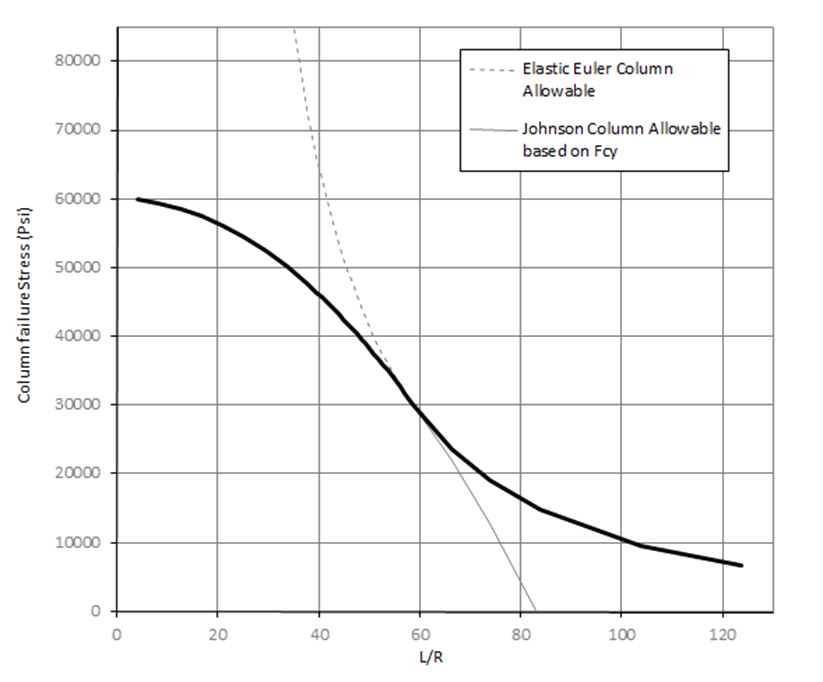### 15.3.2. Johnson-Euler approach for Elastic-Plastic Column Behavior

Reference: Abbott, Richard. Analysis and Design of Composite and Metallic Flight Vehicle Structures 3 Edition, 2019.

The Johnson approach conservatively predicts column strength for short columns where inelastic behavior can have a significant effect on the column strength.

The Johnson short strut formula:

Comparing the Johnson and the Euler column buckling curves for the same material gives the following result:Figure 15.3.2‑1: Johnson Column and Euler Column Buckling Allowable Curves

The Johnson and the Euler curves intersect at the L/R value of:

The full material range column behavior can be approximated by taking the Johnson curve for effective slenderness ratios less than the intersection point and the Euler curve for effective slenderness ratios less than the intersection point. This produces a curve as follows:Figure 15.3.2‑2: Johnson Column and Euler Column Buckling Allowable Curves Superimposed

For a relatively simple approach to column strength where full elasto-plastic material characteristics are not available it is recommended that the Johnson Fcy limit column strength is used for low slenderness ratios and the Euler column allowable is used for columns with high slenderness ratios. This approach is shown by the heavy line in Figure 15.3.2‑2.

This method is defined in this spreadsheet:

### 15.3.2. Johnson-Euler approach for Elastic-Plastic Column Behavior

Reference: Abbott, Richard. Analysis and Design of Composite and Metallic Flight Vehicle Structures 3 Edition, 2019.

The Johnson approach conservatively predicts column strength for short columns where inelastic behavior can have a significant effect on the column strength.

The Johnson short strut formula:

Comparing the Johnson and the Euler column buckling curves for the same material gives the following result:Figure 15.3.2‑1: Johnson Column and Euler Column Buckling Allowable Curves

The Johnson and the Euler curves intersect at the L/R value of:

The full material range column behavior can be approximated by taking the Johnson curve for effective slenderness ratios less than the intersection point and the Euler curve for effective slenderness ratios less than the intersection point. This produces a curve as follows:Figure 15.3.2‑2: Johnson Column and Euler Column Buckling Allowable Curves Superimposed

For a relatively simple approach to column strength where full elasto-plastic material characteristics are not available it is recommended that the Johnson Fcy limit column strength is used for low slenderness ratios and the Euler column allowable is used for columns with high slenderness ratios. This approach is shown by the heavy line in Figure 15.3.2‑2.

This method is defined in this spreadsheet: